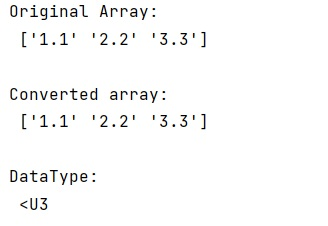# How to convert an array of strings to an array of floats in NumPy?

Learn, how to convert an array of strings to an array of floats in NumPy?
Submitted by Pranit Sharma, on December 24, 2022

NumPy is an abbreviated form of Numerical Python. It is used for different types of scientific operations in python. Numpy is a vast library in python which is used for almost every kind of scientific or mathematical operation. It is itself an array which is a collection of various methods and functions for processing the arrays.

## Converting an array of strings to an array of floats in NumPy

The string is a group of characters, these characters may consist of all the lower case, upper case, and special characters present on the keyboard of a computer system. A string is a data type and the number of characters in a string is known as the length of the string.

Suppose get we are given a NumPy array containing string elements and we need to convert them into float elements.
For this purpose, we will use the astype() method inside which we will pass the np.float as an argument.

Let us understand with the help of an example,

## Python code to convert an array of strings to an array of floats in NumPy

```# Import numpy
import numpy as np

# Creating a numpy array
arr = np.array(['1.1', '2.2', '3.3'])

# Display original array
print("Original Array:\n",arr,"\n")

# Converting type of arr from string to float
res = arr.astype(float)

# Display result
print("Converted array:\n",arr,"\n")

# Display arr data type
print("DataType:\n",arr.dtype)
```

Output: